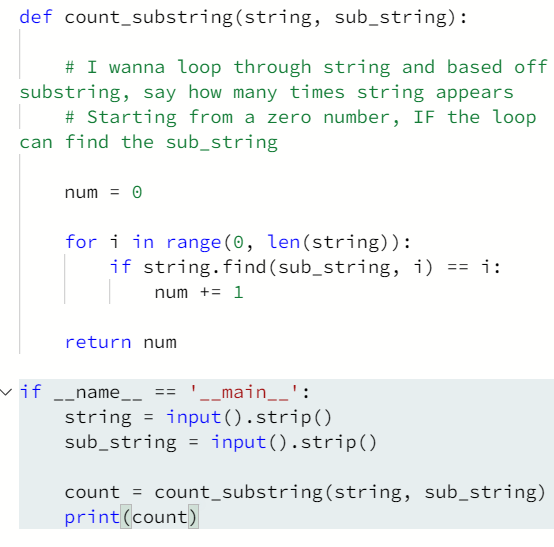# HackerRank – Python – Find a String – Solution

Publish Date - March 1st, 2023

|

Another simpler task if you read the fine print in the question and understand how to do string manipulation in any major coding language (Python, Java, C++, JavaScript, PHP etc..). The question gives you most of the answer VIA the FOR loop and the string manipulation line computer science page. However, you still need to string the logic together to get your answer.

## The problem

In this challenge, the user enters a string and a substring. You have to print the number of times that the substring occurs in the given string. String traversal will take place from left to right, not from right to left.

NOTE: String letters are case-sensitive.

Input Format

The first line of input contains the original string. The next line contains the substring.

Constraints

1 < len(string) < 200
Each character in the string is an ascii character.

Output Format

Output the integer number indicating the total number of occurrences of the substring in the original string.

Sample Input

``````ABCDCDC
CDC
``````

Sample Output

``````2
``````

Concept

Some string processing examples, such as these, might be useful.
There are a couple of new concepts:
In Python, the length of a string is found by the function `len(s)`, where  is the string.
To traverse through the length of a string, use a for loop:

``````for i in range(0, len(s)):
print (s[i])
``````

A range function is used to loop over some length:

``````range (0, 5)
``````

## The solution

The find() function is very useful for string manipulation, and you’ll find it used in a lot of data science / machine learning tools as it’s useful for parsing and aggregating data.

``````def count_substring(string, sub_string):

# I wanna loop through string and based off substring, say how many times string appears
# Starting from a zero number, IF the loop can find the sub_string, increment by 1.

num = 0

for i in range(0, len(string)):
if string.find(sub_string, i) == i:
num += 1

return num

if __name__ == '__main__':
string = input().strip()
sub_string = input().strip()

count = count_substring(string, sub_string)
print(count)``````

The count_substring function is only my code and you can see I wrote the logic down in comments. The solution is pretty simple:

`` num = 0 ``

and then have your FOR loop move through the count of string input starting from 0.

`````` if string.find(sub_string, i) == i:
num += 1 ``````

The conditional above is the important part. You’re telling Python; “Within the string input, if sub_string exists in the looping” increment your number count by 1.

``````if string.find(sub_string) == i:
num += 1 ``````

Note: (See above) it’s important that tell python to start looking for the sub_string at the i. If you don’t the loop, will stop at the first value and always give you 1 as your output. This makes sense if you say it out loud, but won’t give you the right answer.

You could possibly use a doWhile to solve this issue, but why do that when a conditional with a constraint suffices :).

I hope this helps your Python journey!